##### Chemistry Workbook For Dummies with Online Practice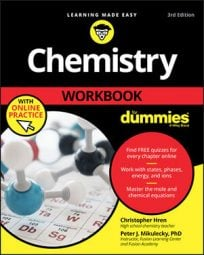Many calculations that you make in chemistry will involve unit conversions (for example, between meters and millimeters, or between meters and inches). Fortunately, you don’t need to know all possible unit conversions.

Instead of memorizing or looking up conversion factors between all types of units, you can memorize just a handful of conversion factors and use them one after another, letting the units guide you each step of the way.

Say you want to know the number of seconds in a standard calendar year (clearly a very large number, so don’t forget about scientific notation). Very few people have this conversion memorized — or will admit to it — but everyone knows that there are 60 seconds in a minute, 60 minutes in an hour, 24 hours in a day, and 365 days in a standard calendar year. So use what you know to get what you want!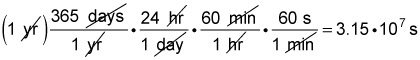Here’s another example: A chemistry student measures a length of 423 mm, yet the lab she’s working on requires that it be in kilometers. What is the length in kilometers?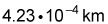## Two-step method

You can go about solving this problem in two ways. You’ll first look at the slightly longer way involving two conversions, followed by a nice, simple one-step problem.

This conversion requires you to move across the metric-system prefixes. When you’re working on a conversion that passes through a base unit, it may be helpful to treat the process as two steps, converting to and from the base unit. In this case, you can convert from millimeters to meters and then from meters to kilometers: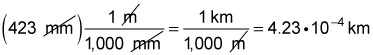You can see how millimeters cancels out, and you’re left with meters. Then meters cancels out, and you’re left with your desired unit, kilometers.

## One-step method

The second way you can approach this problem is to treat the conversion from milli- to kilo- as one big step: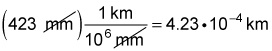Notice the answer doesn’t change; the only difference is the number of steps required to convert the units. You’re simply combining the two denominators in the two-step conversion (1,000 mm and 1,000 m) into one. Rewriting each 1,000 as 10³ may help you see how the denominators combine.